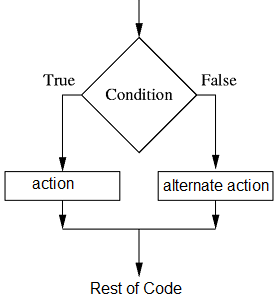VB.Net Tutorial

# How to use IF ELSE in VB.NET

The conditional statement "IF...ELSE" is a fundamental construct in programming that allows for the examination of provided conditions and facilitates decision-making based on those conditions. This statement serves as a crucial tool for controlling the flow of a program based on specific criteria.

When using the IF...ELSE statement, developers can evaluate various conditions by employing comparison operators, such as "equals to" (==), "greater than" (>), "less than" (<), "not equal to" (!=), and others. These operators enable the comparison of values or variables to determine if they meet certain criteria or relationships.

Additionally, logical operators, such as "AND," "OR," and "NOT," can be used within the conditional statement to combine multiple conditions or negate a condition. This allows for more complex and comprehensive evaluations, where multiple conditions need to be satisfied or specific conditions need to be excluded.By utilizing the IF...ELSE statement in combination with comparison and logical operators, developers can effectively analyze data or variables and make informed decisions within their programs. This decision-making process enables the program to dynamically respond to different scenarios or user inputs, resulting in more flexible and adaptive behavior.

The ability to examine conditions and make decisions based on them is a fundamental aspect of programming logic. The IF...ELSE statement, together with comparison and logical operators, empowers developers to incorporate conditional branching and logic into their code, providing a mechanism for implementing different paths or actions based on the evaluation of conditions.

If the contition is TRUE then the control goes to between IF and Else block , that is the program will execute the code between IF and ELSE statements.

If the contition is FLASE then the control goes to between ELSE and END IF block , that is the program will execute the code between ELSE and END IF statements.

If you want o check more than one condition at the same time , you can use ElseIf .

Just take a real-time example - When we want to analyze a mark lists we have to apply some conditions for grading students depends on the marks.

Following are the garding rule of the mark list:

1) If the marks is greater than 80 then the student get higher first class

2) If the marks less than 80 and greater than 60 then the student get first class

3) If the marks less than 60 and greater than 40 then the student get second class

4) The last condition is , if the marks less than 40 then the student fail.

Now here implementing these conditions in a VB.NET program.

1. If totalMarks >= 80 Then 2. MsgBox("Got Higher First Class ") 3. ElseIf totalMarks >= 60 Then 4. MsgBox("Got First Class ") 5. ElseIf totalMarks >= 40 Then 6. MsgBox("Just pass only") 7. Else 8. MsgBox("Failed") 9. End If
1. Line 1 : Checking the total marks greaterthan or equal to 80.
2. Line 2 : If total marks greater than 80 show message - "Got Higher First Class ".
3. Line 3 : Checking the total marks greaterthan or equal to 60.
4. Line 4 : If total marks greater than 60 show message - "Got First Class ".
5. Line 5 : Checking the total marks greaterthan or equal to 40.
6. Line 6 : If total marks greater than 40 show message - "Just pass only".
7. Line 7 : If those three conditions failed program go to the next coding block.
8. Line 8 : If all fail shows message "Failed".
9. Line 9 : Ending the condition block.
VB.NET Source Code
Public Class Form1 Private Sub Button1_Click(ByVal sender As System.Object, ByVal e As System.EventArgs) Handles Button1.Click Dim totalMarks As Integer totalMarks = 59 If totalMarks >= 80 Then MsgBox("Gor Higher First Class ") ElseIf totalMarks >= 60 Then MsgBox("Gor First Class ") ElseIf totalMarks >= 40 Then MsgBox("Just pass only") Else MsgBox("Failed") End If End Sub End Class

In this example the total marks is 59 , when you execute this program you will get in messagebox "Just Pass Only"

If you want to check a condition within condition you can use nested if statements.